# VBA Len Function

What if you are given the sentence “Hello World, VBA is an Excellent Language!!” and you are asked to find out the total number of characters in it? How do you find it? You are not going to find it by calculating it manually.

This tutorial will run you through the definition, syntax, parameters, return type, and different examples explaining how to write a macro code using the VBA LEN function.

## What is VBA LEN Function?

The VBA LEN function is used to return the length of the given string.

The VBA Len considers the variant variable as a string. In other words, the VBA Len Function is used when the user wants to count the number of characters in variables that are defined as strings or variants. Suppose you declare the VBA Len function using other variables, unlike integer, long, single or double. In that case, this function will return the count of the number of bytes needed to store the supplied variable.

Many users confuse the VBA Len function with the Excel worksheet function. Since it is an inbuilt function for both worksheet and VBA, the syntax, the arguments, and the output (both return the length of a string) are also the same for both platforms. As a VBA function, you can use the LEN in macro that you can code using the MS Visual Basic Editor.

### Parameters

Expression (required)– This parameter represents the string or variable for which you want to calculate the length.

### Return

If the Expression is of String or variant data type, the VBA Len function returns the count of characters present in the given supplied string else for any other data type (integer, long, single or double) it the count of the number of bytes needed to store the variable.

## Examples

### # LEN Function Example 1: Extract the length of the string “Reema Panda”.

VBA LEN is the most used string function. It quickly returns the length of the string or the characters present in the supplied value. Therefore, it helps the users in various logics.

Follow the step-by-step guidance to extract the length of the string using the VBA LEN function in Microsoft Visual Basic Editor:

Step 1: Go to your Excel workbook. Open the Visual Basic window either by clicking on developer window -> visual basic editor or directly click on the shortcut keywords Alt +F11.

Step 2: The VB Editor window will be displayed. The next step is to create a module. From the ribbon tab click on Insert-> Click on Module.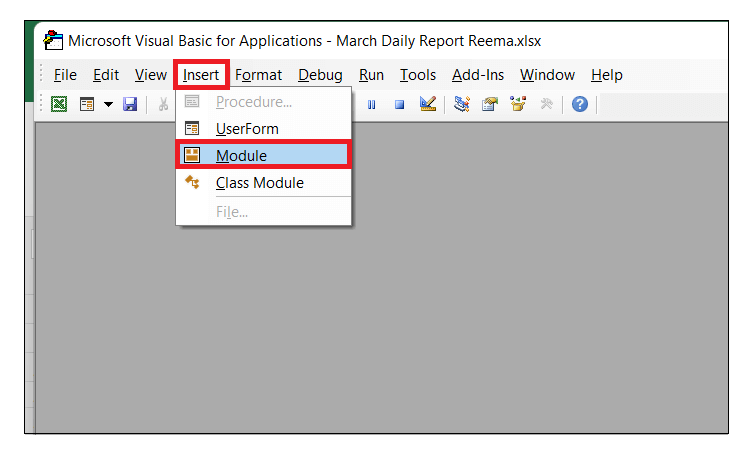Step 3: A module will be inserted. We will write our macro in this module window only. Start the program by introducing the name of your program followed by the declaration of the variable.

Refer to the below given macro code:

Step 4: Next, we will calculate the String length using the VBA LEN function. Further in the function, it will ask you for the Expression. We will pass the String, i.e., ‘Reema Panda”.

Next, we will store the fetch value in our variable and display the function’s output using MsgBox.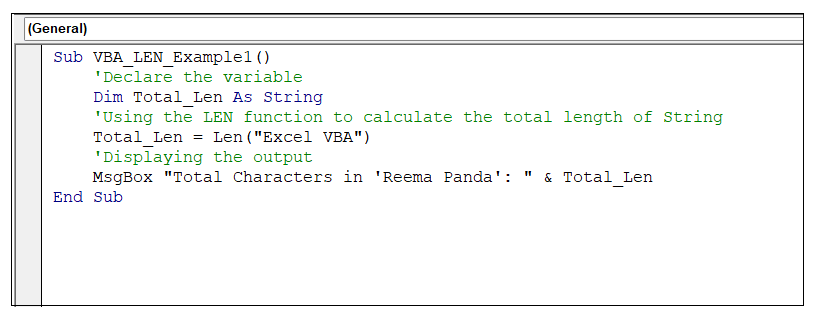Output

Run your macro either by clicking Run or by pressing the F5 key. You will have the following output.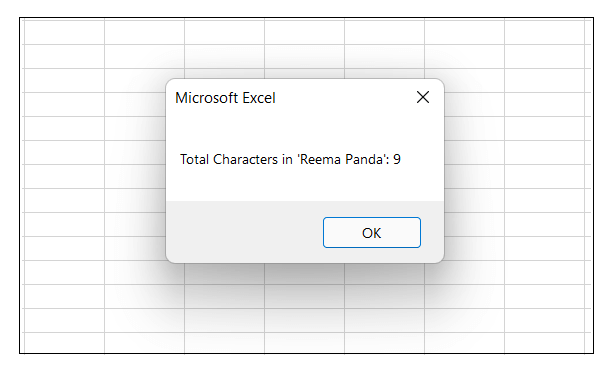As a result, in the above image, you will notice that VBA has returned the length of the string as 9. It has also considered the space as a single character.

### #LEFT Function Example 2: Extract the employee data and Remarks Separately (from below given data table)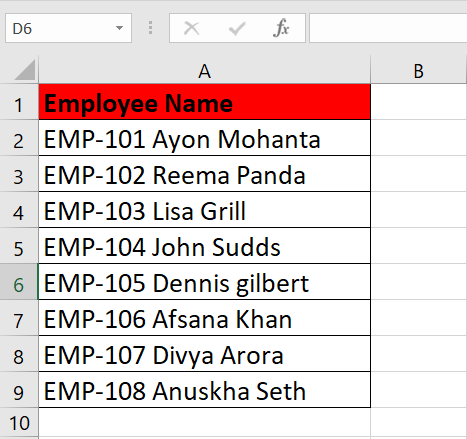The purpose of the VBA LEN function is mostly fulfilled when combined with other functions. Though, for Example1, we have used the LEN function directly, that”s not how it”s used. To solve the above example, we will be using the combination of LEN, RIGHT & INSTR functions.

Below given are the steps to write a VBA macro to extract the employee data and remarks separately from the above table using the VBA LEN function:

Step 1: Open the VBA developer tab. Go to Excel worksheet, from the ribbon tab click on developer window -> visual basic editor or directly click on the shortcut keywords Alt +F11.

Step 2: The VB Editor window will be displayed. The next step is to create a module. From the ribbon tab click on Insert-> Click on Module.Step 3: A module will be inserted. We will write our macro in this module window only. Start the program by introducing the name of your program followed by the declaration of the variable.

Refer to the below given macro code:

Step 4: Since we have to apply the function for a range of cells in Excel so we will use the FOR loop to repeat the logic for all cells. In the For loop, do the following:

• In the val variable we will store the value of the activecell data.
• Using the LEFT and TRIM function, we will calculate the leftmost value (employee id) of the string. Will store the fetched value in column 2.
• Using the MID , LEN & TRIM function we will fetch the employee name and store the value in third column.

Refer to the below given macro code: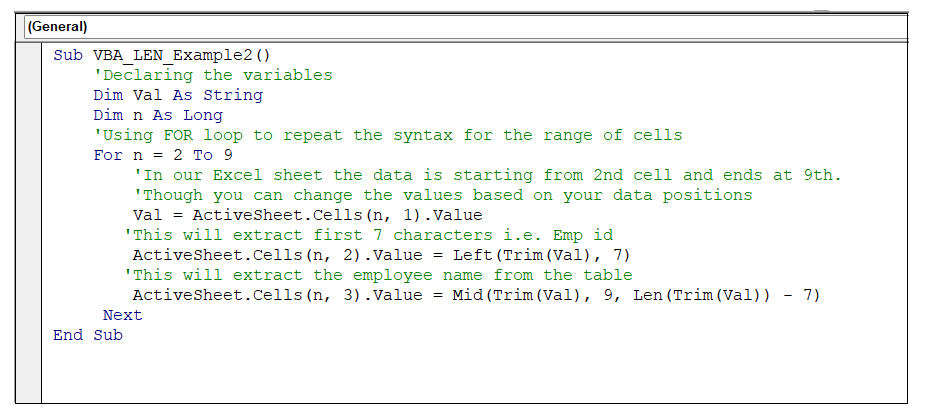Output

Run your macro either by clicking Run or by pressing the F5 key. You will have the following output.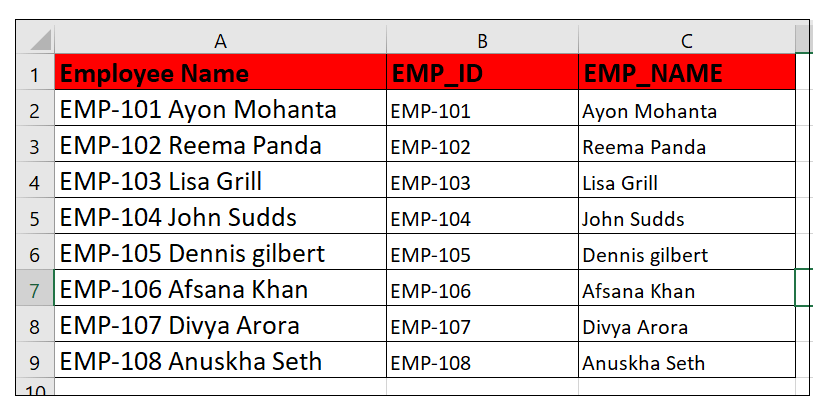As soon as, you run the program you will notice the EMP_ID and EMP_Name column will be automatically filled with their respective data.

### #LEFT Function Example 3: Extract the last name of an employee using VBA LEN function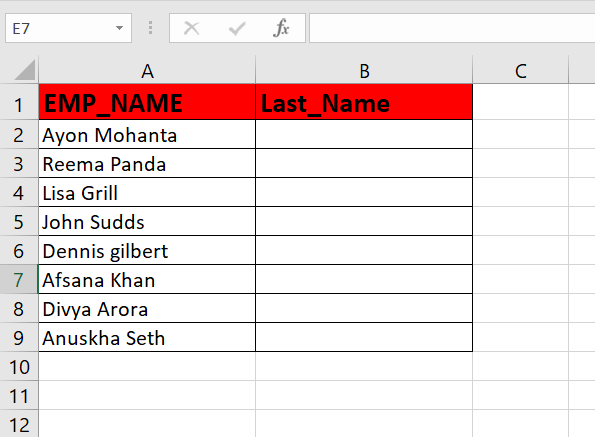In Excel, fetching the last name from the employee table is one of the common operations. However, most people use the RIGHT function to fetch the last names. But if you want to run a dynamic program for all your cells, the standalone RIGHT function won”t work. Similarly, in this example, we will be using a combination of RIGHT, LEN, and INSTR functions to fetch the last name form the above table dynamically.

Follow the step-by-step guidance to extract the last name from the above Excel Employee name table:

Step 1: Go to your Excel workbook. Open the Visual Basic window either by clicking on developer window -> visual basic editor or directly click on the shortcut keywords Alt +F11.

Step 2: The VB Editor window will be displayed. The next step is to create a module. From the ribbon tab click on Insert-> Click on Module.Step 3: A module will be inserted. We will write our macro in this module window only. Start the program by introducing the name of your program followed by the declaration of the variable.

Refer to the below given macro code:

Step 4: Since we have to find the last name dynamically for a range of cells therefore we will use the FOR loop to repeat the logic for all cells. In the For loop, do the following:

• In the LstName variable we will store the value of the activecell data.
• Using the RIGHT function, we will calculate the leftmost value (employee id) of the string.
• Using the LEN function to calculate the count of characters of the string
• Using the INSTR function to calculate the space character.

Refer to the below given macro code:Output

Run your macro either by clicking Run or by pressing the F5 key. You will have the following output.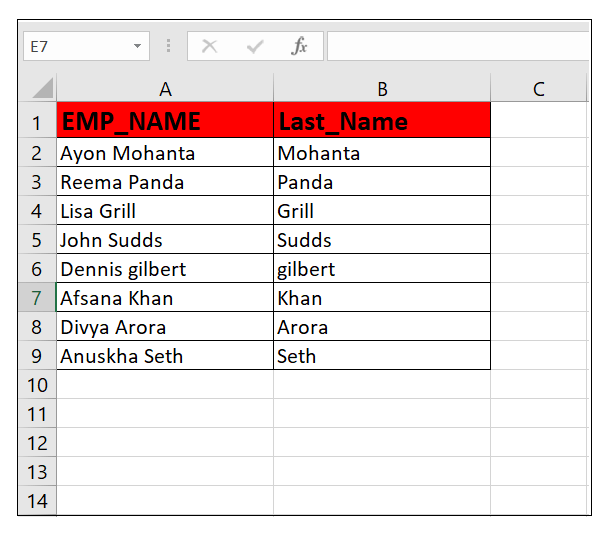As a result, running the above macro code will automatically extract the last names form the employee table.

Next Topic#